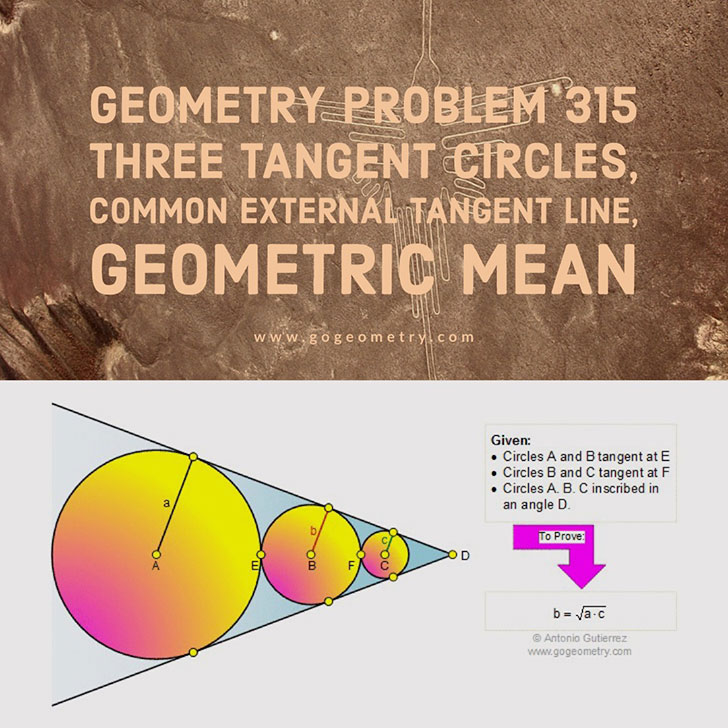# Geometry Problem 315: Three tangent circles, Common external tangent line, Geometric MeanThe figure shows circles A (radius a), B (radius b), and C (radius c) tangent to both sides of an angle D. Circles A and B are tangent at the point E, and circles B and C are tangent at the point F. Prove that b is the geometric mean of a and c. View or post a solution.Home | Geometry | Search | Geometric Mean | Problems | Art | All Problems | 311-320 | Email